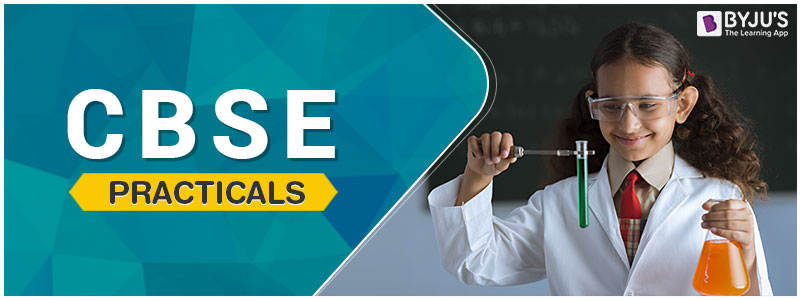# CBSE Practicals for Classes 6 to 12CBSE practical experiments are as important as theoretical knowledge to the students. The human mind learns better when it sees things. Hence, practical knowledge is vital for all students. The practical experiments also help students learn better as they can visualise the concepts and principles. Students must know that CBSE practical exam for Class 11 and Class 12 consists of 30 marks.

For Classes 9 and 10, the practical exam is for 20 marks. As the final exam approaches, students become busy with their exam preparation and ignore preparing for the CBSE practical exams.  But, they must aim to get full marks in the practical exam to increase their overall marks in the final exam. Therefore, students are advised to pay attention to the practical exam and prepare for all the Physics, Chemistry, and Biology experiments and viva questions.

## CBSE Practicals

Click on the links below to get the practical exam experiments for Classes 6 to 8, 9, 10, 11 and 12, Physics, Chemistry and Biology.

### CBSE Practical for Class 6 to 8

CBSE Class 6 to 8 Science Lab Manual
CBSE Class 6 to 8 Maths Lab Manual and Projects

### CBSE Practical for Class 9

CBSE Class 9 Science Practicals and Lab Manual
CBSE Class 9 Maths Practicals, Activities and Lab Manual

### CBSE Practical for Class 10

CBSE Class 10 Science Practicals
CBSE Class 10 Maths Practicals, Activities and Projects

### CBSE Practical for Class 11

CBSE Physics Practical Class 11
CBSE Chemistry Practical Class 11
CBSE Biology Practical Class 11

### CBSE Practical for Class 12

CBSE Physics Practical Class 12
CBSE Chemistry Practical Class 12
CBSE Biology Practical Class 12

### Tips to Prepare for CBSE Practical Exams

Some tips to prepare for the CBSE practical exams are given below.

1. Students must have full knowledge of the theories and principles behind the experiments before performing them.
2. Graphs and formulas are an integral part of the experiments, so students must practise them thoroughly.
3. Students must thoroughly revise the practical experiments and lab manuals before the exam.
4. Practising diagrams can help students visualise and prepare better for the exam.
5. It is very important that students know each and every theory, law, and principle behind the experiment.
6. Students are advised to remain calm and focused while performing the experiment so that they can document the entire procedure.
7. Before the experiments, students must clear all their doubts from their teachers.
8. Important viva questions must be noted and revised properly before the exam.

Students can follow the above-mentioned tips to prepare for their CBSE practical exams. We have provided the CBSE Classes 11 and 12 practical experiment notes, lab manuals, projects, experiments, and activities for Maths, Physics, Chemistry and Biology. It will help students to prepare effectively for their practical exams.

Stay tuned to BYJU’S to get the latest notification on CBSE exams along with the CBSE syllabus, sample papers, marking scheme and more.